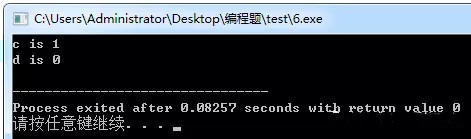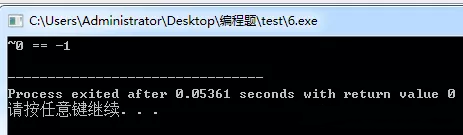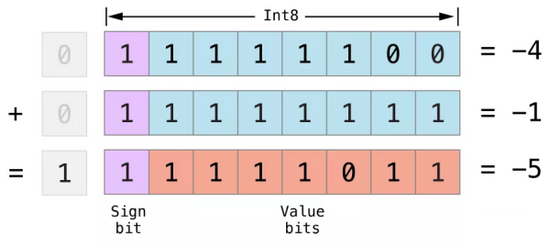# Hey! Don’t forget this C language knowledge~ 0 = = – 1 (problem)

Time：2021-7-29

Today, I’ll take you to review a forgotten C language knowledge point, the storage of negative numbers and a typical investigation form.

# Let’s look at a topic first

#include

int main()

int   a,b,c,d;    // perhaps     int   a,b,c,d;

a=0x8;

b=a>>1;

c=~(~0<<1);

d=b&c;

printf(“c is %d\n”,c);

printf(“d is %d\n”,d);# Resolution:

Suppose the computer is storing eight bits

The storage of 0 is     0b   0000   0000

~Bitwise inversion     0b   one thousand one hundred and eleven   one thousand one hundred and eleven

Shift left one bit      0b   one thousand one hundred and eleven   one thousand one hundred and ten       Fill 0 in neutral

Then reverse by bit    0b   0000   0001

So C is equal to 1

Here is a step-by-step derivation. You will ignore a key process, that is, ~0 what you calculate is 0b 1111 1111, a large negative number, which is contrary to the common sense you think. We will discuss it below.

# Another example

#include

int main()

printf(“~0 == %d\n”, ~0);The storage of 0 is     0b   0000   0000

~Bitwise inversion     0b   one thousand one hundred and eleven   one thousand one hundred and eleven

This topic is relatively straightforward. It is ~ 0 = = – 1 directly. Sometimes we don’t understand why 0b 1111 represents – 1 in memory, because it is a large negative number anyway.

But actually,Negative numbers are stored in memory in the form of complementsIn other words, 0b 1111 1111 is a complement, so its inverse code is 0b 1111 1110, and the original code is 0b 1000 0001, that is – 1 (note that the sign bit remains unchanged when negative numbers are used for inverse complement)

# conclusion

0b 1111 1111 == -1 （~0）

0b 1111 1110 == -2 （~1）

0b 1111 1101 == -3 （~2）

You can think of it as a formula ~ a = = – [a + 1]

If you encounter a bottleneck in learning programming, feel confused and confused,Attention【Up home page】JoinC language c + + Learning Circle

Learning exchange, technical discussion, interview guidance, resume modification… There are too many source materials and other learning materials, zero basic videos waiting for you!

# Supplementary notes

Why should integers be stored in memory as complements. Because the original code of positive numbers and the complement of negative numbers are the same, we mainly discuss what negative numbers are stored in memory in the form of complement.

The reasons or advantages of using complement are as follows. The use of complement operation has the following two characteristics:

1) Because the sign bit and other bits can be processed uniformly by using complement. At the same time, subtraction can also be processed by addition, that is, if it is a number represented by complement, whether it is addition or subtraction, it can be realized directly by addition.

2) When two numbers represented by complement are added, if the highest bit (sign bit) has carry, the carry is discarded.

Such an operation has two advantages:

1) The sign bit can participate in the operation together with the valid value part, so as to simplify the operation rules. Thus, the structure of the arithmetic unit can be simplified and the operation speed can be improved（ Subtraction can be expressed by addition)

2) Addition is easier to implement than subtraction. The subtraction operation is converted into addition operation, which further simplifies the circuit design of the arithmetic unit in the computer.

The following figure best illustrates why the subtraction of integers (i.e. the operation of negative numbers) can be changed into addition with complement:# In depth proof:

The result of multiplication and division with the original code with signed bits is correct, but there are problems in addition and subtraction, as follows: suppose the word length is 8 bits

( 1 ) – ( 1 ) = ( 1 ) + ( -1 ) = ( 0 )

It can be expressed as:

(00000001) original  + ( 10000001) original  = ( 10000001) original  = ( – two  )  Obviously not.

Because there is no problem in the addition operation of two integers, it is found that the problem occurs in the negative number with signed bits, and the bit by bit inversion of other bits except signed bits produces inverse code. The value space of the inverse code is the same as that of the original code and corresponds one by one. The following is the subtraction operation of inverse code:

( 1 ) – ( 1 ) = ( 1 ) + ( -1 ) = ( 0 )

It can be expressed as:

(00000001)   Counter+  ( 11111110) reverse  = ( 11111111) reverse  = ( – 0  )  something the matter.

( 1 ) – ( 2) = ( 1 ) + ( -2 ) = ( -1 )

It can be expressed as:

(00000001)   Counter+  ( 11111101) reverse  = ( 11111110) reverse  = ( – one  )  correct

The problem is (+ 0) and (- 0). In people’s calculation concept, zero is not positive or negative.

Therefore, the concept of complement is introduced. The complement of a negative number is to add one to the inverse code, while the positive number remains the same. The original code and inverse complement of a positive number are the same. (- 0) is replaced by (- 128) in the complement, so the representation range of complement is:

(- 128 ~ 127) 256 in total.

Note: (- 128) has no corresponding original code and inverse code, and the addition and subtraction of (- 128) = (10000000) complement is as follows:

( 1 ) – ( 1 ) = ( 1 ) + ( -1 ) = ( 0 )

It can be expressed as:

(00000001) supplement  + ( 11111111) supplement  = ( (00000000) supplement  = (  0  )  correct

( 1 ) – ( 2) = ( 1 ) + ( -2 ) = ( -1 )

It can be expressed as:

(00000001)   Supplement+  ( 11111110)   Supplement=  ( 11111111) supplement  = ( – one  )  correct

Another reason for using complement representation is to prevent the number of machines with 0 from having two codes. The original code and the inverse code represent 0 in two forms + 0 and – 0, and we know that + 0 and – 0 are the same. In this way, the range of integers represented by 8-bit original code and inverse code is – 127-127 (11111111-01111111), and when expressed by complement, 00000000 is + 0, i.e. 0; 10000000 is no longer – 0, but – 128. In this way, the range of numbers represented by complement is – 128 ~ + 127, which not only increases the representation range of a number, but also ensures the uniqueness of 0 coding.

Why is the inverse code and complement of a positive number the same as the original code? This is a rule or an agreement. It doesn’t make much sense. You can’t calculate it.

Complement is just a way to think of negative numbers. The purpose is subtraction and addition. Subtraction can be realized by adding complement. The complement can be obtained by adding 1 to the inverse code. So Tao has the inverse code of negative numbers.

There is a hardware “adder” in the computer. With complement, subtraction can also be done with adder. In the computer, the computing speed of hardware is much faster than that of software. This is the reason for making patterns of inverse code, complement code and original code.

# Image description

The function of introducing complement code is to make it easier for the computer to perform subtraction. For example, if the time is 12 hours, the current accurate time is 4 o’clock. A table shows 7 o’clock. To calibrate the time, we can turn the clock back 7-4 = 3 grid or forward 12-3 = 9 grid. The subtraction of the computer can be converted into – 3 = + 9, This can simplify the hardware equipment of the computer to do complex subtraction.

Article source:   http://developer.51cto.com/art/202102/644783.htm## VBS obtains the operating system and its version number

VBS obtains the operating system and its version number ? 1 2 3 4 5 6 7 8 9 10 11 12 ‘************************************** ‘*by r05e ‘* operating system and its version number ‘************************************** strComputer = “.” Set objWMIService = GetObject(“winmgmts:” _  & “{impersonationLevel=impersonate}!\\” & strComputer & “\root\cimv2”) Set colOperatingSystems = objWMIService.ExecQuery _  (“Select * from […]